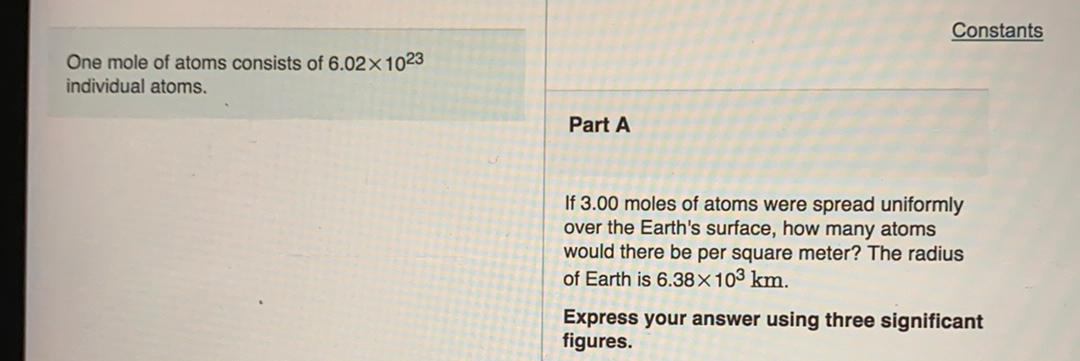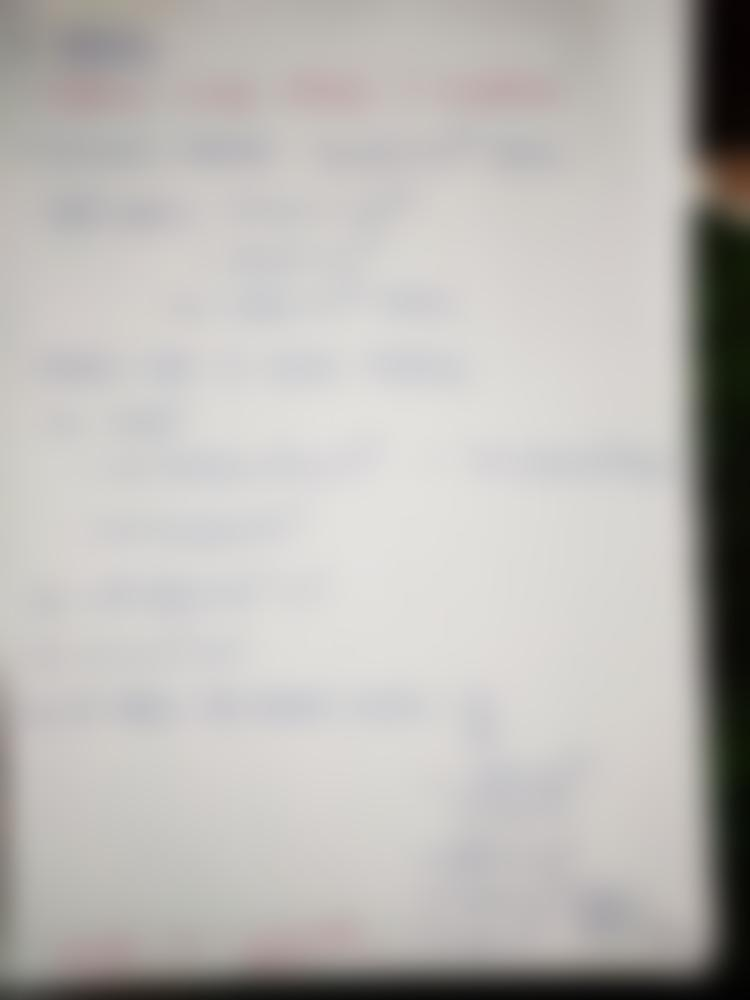Question:

Constants One mole of atoms consists of 6.02 x 1023 individual atoms. Part A If 3.00 moles of atoms were spread uniformly over tConstants One mole of atoms consists of 6.02 x 1023 individual atoms. Part A If 3.00 moles of atoms were spread uniformly over the Earth's surface, how many atoms would there be per square meter? The radius of Earth is 6.38 x 103 km. Express your answer using three significant figures.